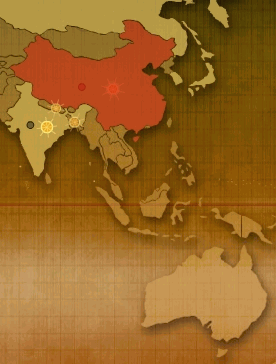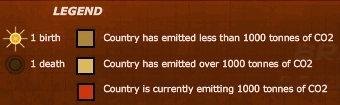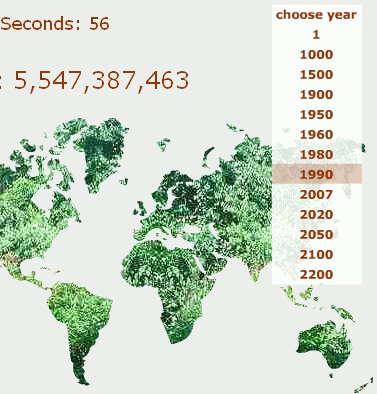Search IntMath
Close

# Breathing Earth - visual display of world polluters

By Murray Bourne, 19 Aug 2007

Breathingearth.net has a nice simulation of the most polluting countries.The legend is as follows:It reminds me of my own World Population simulation, where you can get a sense of population growth in the past, and future.### Comment Preview

HTML: You can use simple tags like <b>, <a href="...">, etc.

To enter math, you can can either:

1. Use simple calculator-like input in the following format (surround your math in backticks, or qq on tablet or phone):
a^2 = sqrt(b^2 + c^2)
(See more on ASCIIMath syntax); or
2. Use simple LaTeX in the following format. Surround your math with $$ and $$.
$$\int g dx = \sqrt{\frac{a}{b}}$$
(This is standard simple LaTeX.)

NOTE: You can mix both types of math entry in your comment.